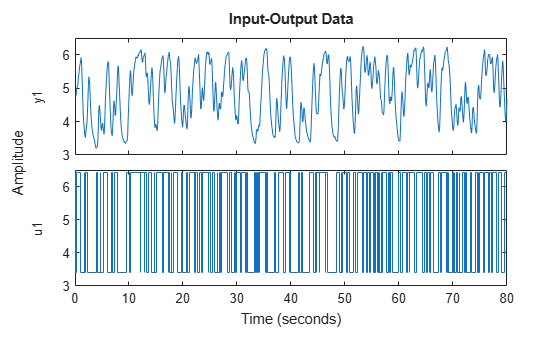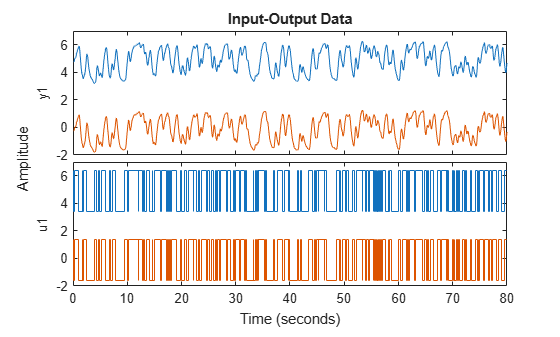Documentation

getTrend

Data offset and trend information

Syntax

T = getTrend(data)
T = getTrend(data,0)
T = getTrend(data,1)

Description

T = getTrend(data) constructs a TrendInfo object to store offset, mean, or linear trend information for detrending or retrending data. You can assign specific offset and slope values to T.

T = getTrend(data,0) computes the means of input and output signals and stores them as InputOffset and OutputOffset properties of T, respectively.

T = getTrend(data,1) computes a best-fit straight line for both input and output signals and stores them as properties of T.

Examples

collapse all

Remove specified offset from input and output signals.

Load SISO data containing vectors u2 and y2.

Create a data object with sample time of 0.08 seconds and plot it.

data = iddata(y2,u2,0.08);
plot(data)The data has a nonzero mean value.

Store the data offset and trend information in a TrendInfo object.

T = getTrend(data);

Assign offset values to the TrendInfo object.

T.InputOffset = 5;
T.OutputOffset = 5;

Subtract offset from the data.

data_d = detrend(data,T);

Plot the detrended data on the same plot.

hold on
plot(data_d)View the mean value removed from the data.

get(T)
ans = struct with fields:
DataName: 'data'
InputOffset: 5
OutputOffset: 5
InputSlope: 0
OutputSlope: 0

Compute input-output signal means, store them, and detrend the data.

Load SISO data containing vectors u2 and y2.

Create a data object with sample time of 0.08 seconds.

data = iddata(y2,u2,0.08);

Compute the mean of the data.

T = getTrend(data,0);

Remove the mean from the data.

data_d = detrend(data,T);

Plot the original and detrended data on the same plot.

plot(data,data_d)